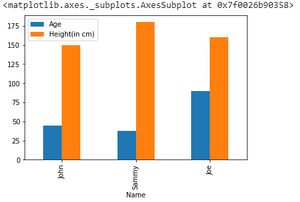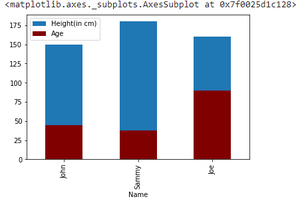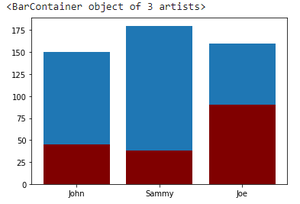GeeksforGeeks App
Open AppBrowser
Continue

# Plot Multiple Columns of Pandas Dataframe on Bar Chart with Matplotlib

Prerequisites:

In this article, we will learn how to plot multiple columns on bar chart using Matplotlib. Bar Plot is used to represent categories of data using rectangular bars. We can plot these bars with overlapping edges or on same axes. Different ways of plotting bar graph in the same chart are using matplotlib and pandas are discussed below.

### Method 1: Providing multiple columns in y parameter

The trick here is to pass all the data that has to be plotted together as a value to ‘y’ parameter of plot function.

Syntax:

matplotlib.pyplot.plot(\*args, scalex=True, scaley=True, data=None, \*\*kwargs)

Approach:

• Import module
• Pass data to plot()
• Plot graph

Example:

## Python3

 `# importing pandas library``import` `pandas as pd``# import matplotlib library``import` `matplotlib.pyplot as plt`` ` `# creating dataframe``df ``=` `pd.DataFrame({``    ``'Name'``: [``'John'``, ``'Sammy'``, ``'Joe'``],``    ``'Age'``: [``45``, ``38``, ``90``],``    ``'Height(in cm)'``: [``150``, ``180``, ``160``]``})`` ` `# plotting graph``df.plot(x``=``"Name"``, y``=``[``"Age"``, ``"Height(in cm)"``], kind``=``"bar"``)`

Output:### Method 2: By plotting on the same axis

Plotting all separate graph on the same axes, differentiated by color can be one alternative. Here again plot() function is employed.

Approach:

• Import module
• Plot first graph
• Plot all other graphs on the same axes

Example:

## Python3

 `# importing pandas library``import` `pandas as pd``# import matplotlib library``import` `matplotlib.pyplot as plt`` ` `# creating dataframe``df ``=` `pd.DataFrame({``    ``'Name'``: [``'John'``, ``'Sammy'``, ``'Joe'``],``    ``'Age'``: [``45``, ``38``, ``90``],``    ``'Height(in cm)'``: [``150``, ``180``, ``160``]``})`` ` `# plotting Height``ax ``=` `df.plot(x``=``"Name"``, y``=``"Height(in cm)"``, kind``=``"bar"``)``# plotting age on the same axis``df.plot(x``=``"Name"``, y``=``"Age"``, kind``=``"bar"``, ax``=``ax, color``=``"maroon"``)`

Output:### Method 3: By creating subplots

Another way of creating such a functionality can be plotting multiple subplots and displaying them as one. This can be done using subplot() function.

Syntax:

subplot(nrows, ncols, index, **kwargs)

Approach:

• Import module
• Create multiple subplots
• Plot on single axes

Example:

## Python3

 `# importing pandas library``import` `pandas as pd``# import matplotlib library``import` `matplotlib.pyplot as plt`` ` `# creating dataframe``df ``=` `pd.DataFrame({``    ``'Name'``: [``'John'``, ``'Sammy'``, ``'Joe'``],``    ``'Age'``: [``45``, ``38``, ``90``],``    ``'Height(in cm)'``: [``150``, ``180``, ``160``]``})`` ` `# creating subplots and plotting them together``ax ``=` `plt.subplot()``ax.bar(df[``"Name"``], df[``"Height(in cm)"``])``ax.bar(df[``"Name"``], df[``"Age"``], color``=``"maroon"``)`

Output:My Personal Notes arrow_drop_up